Function Repository Resource:

# NagelPoint

Get the Nagel point of a triangle

Contributed by: Shenghui Yang
 ResourceFunction["NagelPoint"][{p1,p2,p3}] returns the Nagel point of the triangle defined by vertices p1,p2 and p3.

## Details

The Nagel point of a triangle is the point of concurrence of the three lines that respectively pass through a vertex of a triangle and the corresponding excircle contact point (extouch point) of the opposite side.
ResourceFunction["NagelPoint"] is the isotomic conjugate of the Gergonne point.
The existence of the Nagel point follows directly from Ceva's theorem and the semiperimeter partition of the extouch point.
ResourceFunction["NagelPoint"] effectively uses the triangle center function with a barycentric weighted average of the vertices of the given triangle.
ResourceFunction["NagelPoint"][Triangle[{p1,p2,p3}]] is equivalent to ResourceFunction["NagelPoint"][{p1,p2,p3}].

## Examples

### Basic Examples (1)

Find the Nagel point of three triangle vertices:

 In:=Out=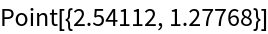In:=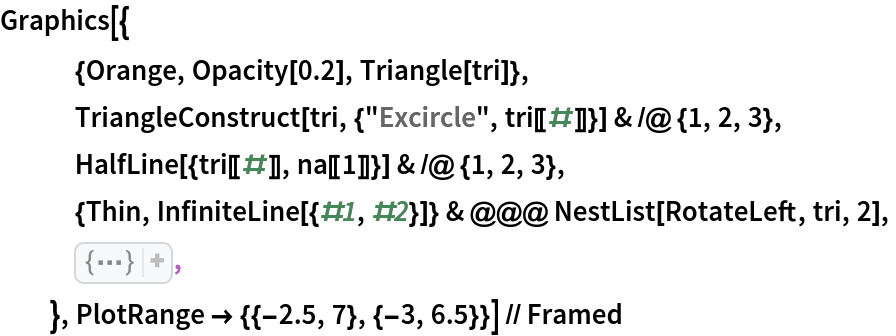Out=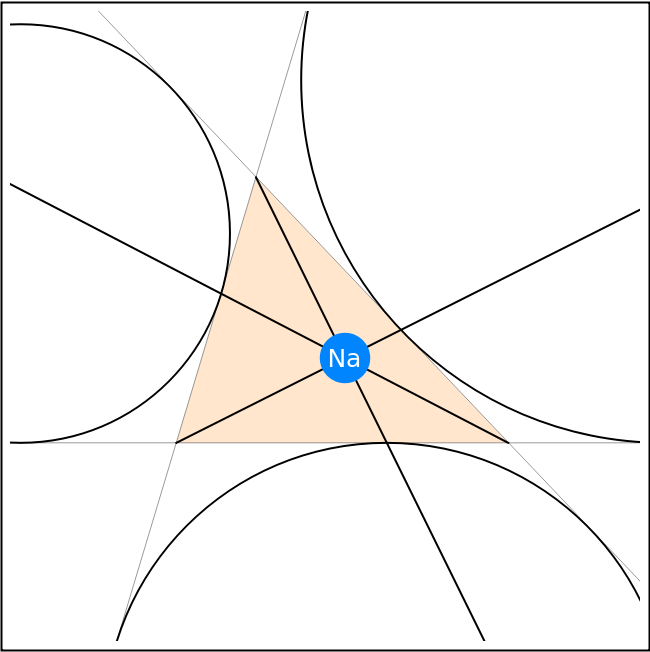### Scope (2)

Compute the Nagel point of a 3D triangle:

 In:=Out=Show the Nagel point and the triangle together:

 In:=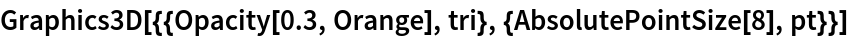Out=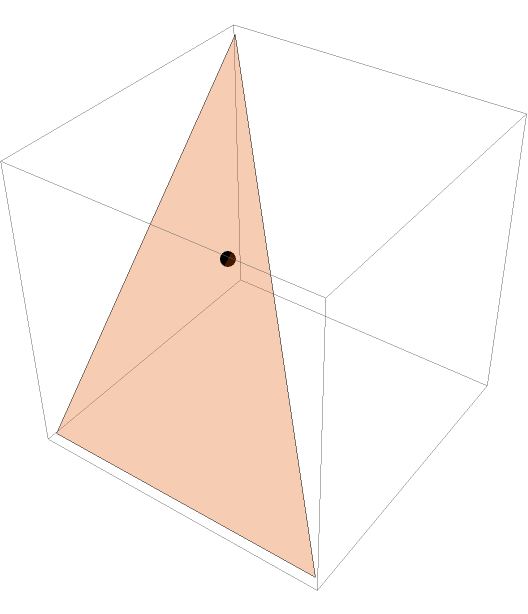### Neat Examples (2)

The Nagel point of the medial triangle is the same as the incenter of the reference triangle:

 In:=Out=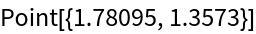In:=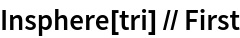Out=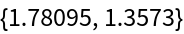In:=Out=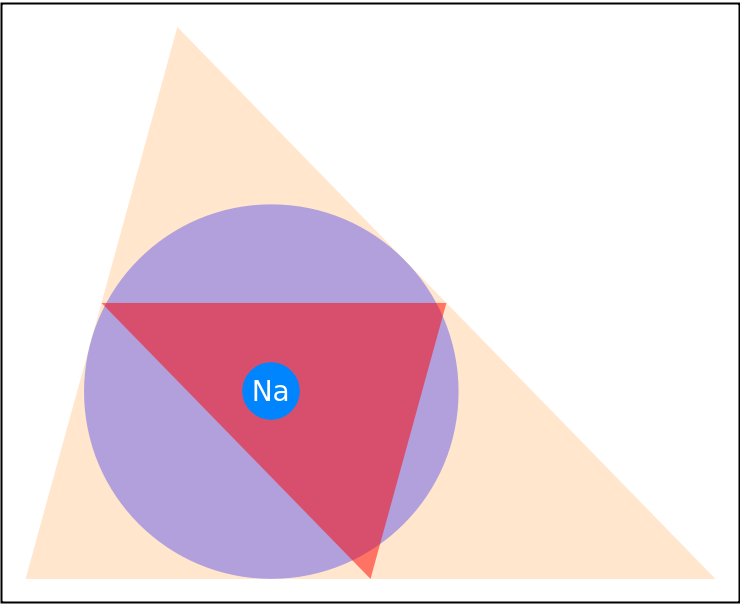The Nagel point, the centroid and the incenter are collinear:In:=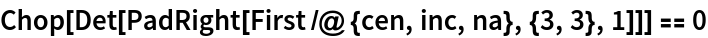Out=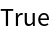In:=Out=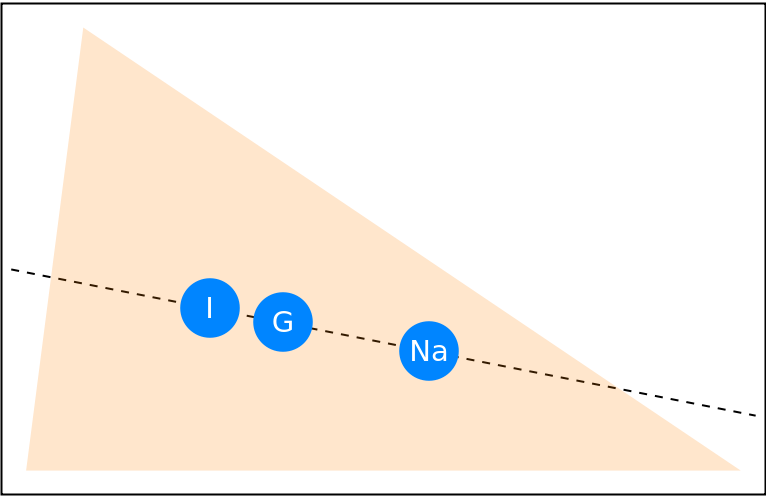Shenghui Yang

## Version History

• 1.0.0 – 01 September 2021